### The Elements Of Geometry Class 9th Mathematics AP Board Solution

##### Question 1.Answer the following:i. How many dimensions a solid has?ii. How many books are there in Euclid’s Elements?iii. Write the number of faces of a cube and cuboid.iv. What is sum of interior angles of a triangle?v. Write three un-defined terms of geometry.Answer:i. A solid has 3 dimensions, namely length, breadth and height.ii. Euclid of Alexandria in Egypt wrote 13 books called ‘The Elements’.iii. A cube is also a cuboid having 6 faces. Hence, both cube and cuboid have 6 faces.iv. By equivalent of fifth postulate, we know that the sum of angle of a triangle is a constant and is equal to two right angles.∴ Sum of interior angles of a triangle = 180°v. In geometry, a point, a line and a plane are the un-defined terms.Question 2.State whether the following statements are true or false? Also give reasons for your answers.a) Only one line can pass through a given point.b) All right angles are equal.c) Circles with same radii are equal.d) A finite line can be extended on its both sides endlessly to get a straight line.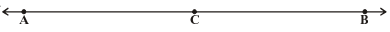e) From the figure, AB > ACAnswer:a) FalseIt is false because through a given point, infinite number of lines can pass.b) True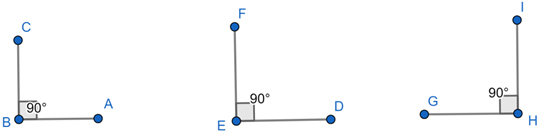Let us consider ∠ABC = 90°, ∠DEF = 90° and ∠GHI = 90°.Here, ∠ABC = ∠DEF = ∠GHIi.e. all the right angles are equal (By Postulate 4).c) TrueTwo circles coincide if we superimpose the region bounded by one circle on the other circle. Then, their centers and boundaries also coincide.∴ Circle with same radii will be equal.d) TrueIt is true by Euclid’s postulate 2.e) TrueC is the midpoint of line segment AB dividing the lines into two halves i.e. AC = BC⇒ AB = AC + BC∴ AB > ACQuestion 3.In the figure given below, show that length AH > AB + BC + CD.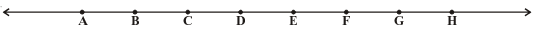Answer:Given figure is drawn above.By Euclid’s Axiom 5,We know that ‘The whole is greater than the part’.In the figure, AH is whole.AB, BC, CD are parts.⇒ AB + BC + CD is also a part.∴ AH > AB + BC + CDQuestion 4.If a point Q lies between two points P and R such that PQ = QR, prove that PQ = 1/2 PR.Answer:Given, a point Q lies between 2 points P and R such that PQ = QR.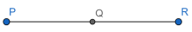Adding PQ on both sides,⇒ PQ + PQ = QR + PQ⇒ 2PQ = PR∴ PQ = 1/2 PRHence proved.Question 5.Draw an equilateral triangle whose sides are 5.2 cm each.Answer:Step 1:Draw a line segment AB of length 5.2 cm.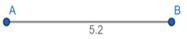Step2:From Euclid’s third postulate, we know that we can draw a circle with any center and any radius.Draw a circle with center A and radius AB.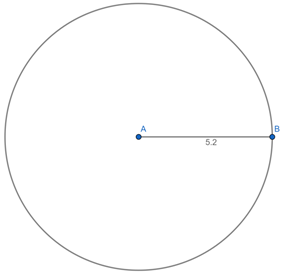Step 3:Draw another circle with center B and radius BA.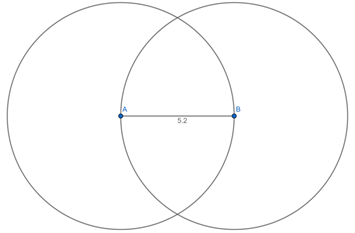Step 4:The two circles meet at C. Join C to A and B to form ΔABC.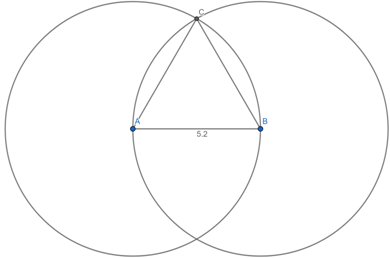Thus, the required equilateral triangle is constructed.Question 6.What is a conjecture? Give an example for it.Answer:Sometimes a certain statement that we think is to be true but that is an educated guess based on observations. Such statements which are neither proved nor disproved are called conjectures (hypothesis). Mathematical discoveries often start out as conjectures.Example: “Every even number greater than 4 can be written as sum of two primes” is a conjecture stated by Gold Bach.Question 7.Mark two points P and Q. Draw a line through P and Q. Now how many lines are parallel to PQ, can you draw?Answer:Let us mark 2 points P and Q. Then, draw a line through P and Q.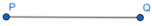There can be infinite number of parallel lines to PQ. So, we cannot draw all of them.Question 8.In the adjacent figure, a line n falls on lines l and m such that the sum of the interior angles 1 and 2 is less than 180°, then what can you say about lines l and m.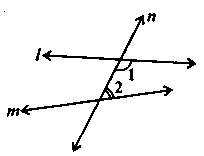Answer:By Euclid’s Postulate 5,We know that if a straight line falling on two straight lines makes the interior angles on the same side of it taken together less than two right angles, then the two straight lines, if produced indefinitely, meet on that side on which the sum of angles is less than two right angles.Given, a line n falls on lines l and m such that the sum of the interior angles 1 and 2 < 180° on the right side of line n.So, the lines l and m will eventually intersect on the right side of line n.Question 9.In the adjacent figure, if ∠1 = ∠3, ∠2 = ∠4 and ∠3 = ∠4, write the relation between∠1 and ∠2 using a Euclid’s postulate.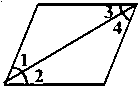Answer:Given,∠1 = ∠3, ∠3 = ∠4 … (i)And ∠2 = ∠4 … (ii)By Euclid’s axiom 1,We know that things which are equal to the same things are equal to one another.∴ From equation (i), ∠1 = ∠4 … (iii)Then, from equations (ii) and (iii) and by axiom 1,⇒ ∠1 = ∠2Question 10.In the adjacent figure, we have BX = 1/2 AB, BY = 1/2 BC and AB = BC. Show that BX = BY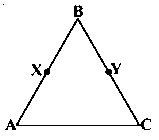Answer:Given, AB = BC … (i)And BX = 1/2 AB and BY = 1/2 BC … (ii)By Euclid’s axiom 7,We know that things which are halves of the same thing are equal to one another.From (i) and (ii),∴ BX = BY

PDF FILE TO YOUR EMAIL IMMEDIATELY PURCHASE NOTES & PAPER SOLUTION. @ Rs. 50/- each (GST extra)

HINDI ENTIRE PAPER SOLUTION

MARATHI PAPER SOLUTION

SSC MATHS I PAPER SOLUTION

SSC MATHS II PAPER SOLUTION

SSC SCIENCE I PAPER SOLUTION

SSC SCIENCE II PAPER SOLUTION

SSC ENGLISH PAPER SOLUTION

SSC & HSC ENGLISH WRITING SKILL

HSC ACCOUNTS NOTES

HSC OCM NOTES

HSC ECONOMICS NOTES

HSC SECRETARIAL PRACTICE NOTES

# 2019 Board Paper Solution

HSC ENGLISH SET A 2019 21st February, 2019

HSC ENGLISH SET B 2019 21st February, 2019

HSC ENGLISH SET C 2019 21st February, 2019

HSC ENGLISH SET D 2019 21st February, 2019

SECRETARIAL PRACTICE (S.P) 2019 25th February, 2019

HSC XII PHYSICS 2019 25th February, 2019

CHEMISTRY XII HSC SOLUTION 27th, February, 2019

OCM PAPER SOLUTION 2019 27th, February, 2019

HSC MATHS PAPER SOLUTION COMMERCE, 2nd March, 2019

HSC MATHS PAPER SOLUTION SCIENCE 2nd, March, 2019

SSC ENGLISH STD 10 5TH MARCH, 2019.

HSC XII ACCOUNTS 2019 6th March, 2019

HSC XII BIOLOGY 2019 6TH March, 2019

HSC XII ECONOMICS 9Th March 2019

SSC Maths I March 2019 Solution 10th Standard11th, March, 2019

SSC MATHS II MARCH 2019 SOLUTION 10TH STD.13th March, 2019

SSC SCIENCE I MARCH 2019 SOLUTION 10TH STD. 15th March, 2019.

SSC SCIENCE II MARCH 2019 SOLUTION 10TH STD. 18th March, 2019.

SSC SOCIAL SCIENCE I MARCH 2019 SOLUTION20th March, 2019

SSC SOCIAL SCIENCE II MARCH 2019 SOLUTION, 22nd March, 2019

XII CBSE - BOARD - MARCH - 2019 ENGLISH - QP + SOLUTIONS, 2nd March, 2019

# HSCMaharashtraBoardPapers2020

(Std 12th English Medium)

HSC ECONOMICS MARCH 2020

HSC OCM MARCH 2020

HSC ACCOUNTS MARCH 2020

HSC S.P. MARCH 2020

HSC ENGLISH MARCH 2020

HSC HINDI MARCH 2020

HSC MARATHI MARCH 2020

HSC MATHS MARCH 2020

# SSCMaharashtraBoardPapers2020

(Std 10th English Medium)

English MARCH 2020

HindI MARCH 2020

Hindi (Composite) MARCH 2020

Marathi MARCH 2020

Mathematics (Paper 1) MARCH 2020

Mathematics (Paper 2) MARCH 2020

Sanskrit MARCH 2020

Sanskrit (Composite) MARCH 2020

Science (Paper 1) MARCH 2020

Science (Paper 2)

Geography Model Set 1 2020-2021

MUST REMEMBER THINGS on the day of Exam

Are you prepared? for English Grammar in Board Exam.

Paper Presentation In Board Exam

How to Score Good Marks in SSC Board Exams

Tips To Score More Than 90% Marks In 12th Board Exam

How to write English exams?

How to prepare for board exam when less time is left

How to memorise what you learn for board exam

No. 1 Simple Hack, you can try out, in preparing for Board Exam

How to Study for CBSE Class 10 Board Exams Subject Wise Tips?

JEE Main 2020 Registration Process – Exam Pattern & Important Dates

NEET UG 2020 Registration Process Exam Pattern & Important Dates

How can One Prepare for two Competitive Exams at the same time?

8 Proven Tips to Handle Anxiety before Exams!